1. /
2. CBSE
3. /
4. Class 07
5. /
6. Mathematics
7. /
8. NCERT Solutions for Class...

# NCERT Solutions for Class 7 Maths Exercise 11.4### myCBSEguide App

Download the app to get CBSE Sample Papers 2023-24, NCERT Solutions (Revised), Most Important Questions, Previous Year Question Bank, Mock Tests, and Detailed Notes.

NCERT solutions for Maths Perimeter and Area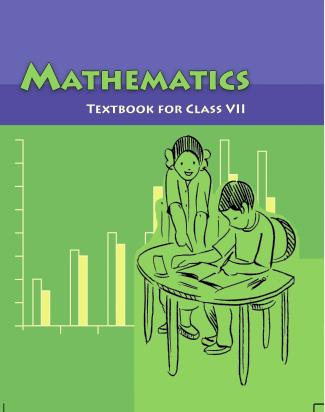## NCERT Solutions for Class 7 Maths Perimeter and Area

###### Question 1.A garden is 90 m long and 75 m broad. A path 5 m wide is to be built outside and around it. Find the area of the path. Also find the area of the garden in hectares.

Length of rectangular garden = 90 m and breadth of rectangular garden = 75 m

Outer length of rectangular garden with path

= 90 + 5 + 5 = 100 m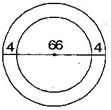Outer breadth of rectangular garden with path

= 75 + 5 + 5 = 85 m

Outer area of rectangular garden with path

= length x breadth = 100 x 85 = 8,500 {tex}{m^2}{/tex}

Inner area of garden without path = length x breadth = 90 x 75 = 6,750 {tex}{m^2}{/tex}

Now Area of path = Area of garden with path – Area of garden without path

= 8,500 – 6,750

= 1,750{tex}{m^2}{/tex}

Since, 1 m2 = {tex}\frac{1}{{10000}}{/tex} hectares

Therefore, 6,750 {tex}{m^2}{/tex}= {tex}\frac{{6750}}{{10000}}{/tex} = 0.675 hectares

NCERT Solutions for Class 7 Maths Exercise 11.4

###### Question 2.A 3 m wide path runs outside and around a rectangular park of length 125 m and breadth 65 m. Find the area of the path.

Length of rectangular park = 125 m, breadth of rectangular park = 65 m and width of the path = 3 m

Length of rectangular park with path

= 125 + 3 + 3 = 131 m

Breadth of rectangular park with path

= 65 + 3 + 3 = 71 m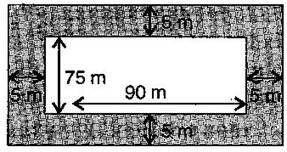{tex}\therefore {/tex} Area of path

= Area of park with path – Area of park without path

= (AB x AD) – (EF x EH)

= (131 x 71) – (125 x 65)

= 9301 – 8125

= 1,176{tex}{m^2}{/tex}

Thus, area of path around the park is 1,176{tex}{m^2}{/tex}.

NCERT Solutions for Class 7 Maths Exercise 11.4

###### Question 3.A picture is painted on a cardboard 8 cm long and 5 cm wide such that there is a margin of 1.5 cm along each of its sides. Find the total area of the margin.

Length of painted cardboard = 8 cm and breadth of painted card = 5 cm

Since, there is a margin of 1.5 cm long from each of its side.

Therefore reduced length

= 8 – (1.5 + 1.5) = 8 – 3 = 5 cm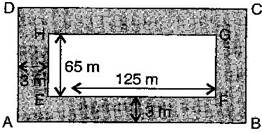= 5 – (1.5 + 1.5) = 5 – 3 = 2 cm

{tex}\therefore {/tex} Area of margin = Area of cardboard (ABCD) – Area of cardboard (EFGH)

= (AB x AD) – (EF x EH)

= (8 x 5) – (5 x 2)

= 40 – 10

= 30 {tex}c{m^2}{/tex}

Thus, the total area of margin is 30 {tex}c{m^2}{/tex}.

NCERT Solutions for Class 7 Maths Exercise 11.4

###### Question 4.A verandah of width 2.25 m is constructed all along outside a room which is 5.5 m long and 4 m wide. Find:

(i) the area of the verandah.

(ii) the cost of cementing the floor of the verandah at the rate of Rs. 200 per {tex}{m^2}{/tex}.

(i) The length of room = 5.5 m and width of the

room = 4 m

The length of room with verandah

= 5.5 + 2.25 + 2.25 = 10 m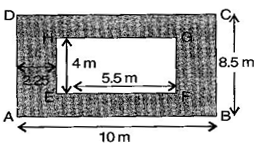The width of room with verandah

= 4 + 2.25 + 2.25 = 8.5 m

Area of verandah

= Area of room with verandah – Area of room without verandah

= Area of ABCD – Area of EFGH

= (AB x AD) – (EF x EH)

= (10 x 8.5) – (5.5 x 4)

= 85 – 22

= 63 {tex}{m^2}{/tex}

(ii) The cost of cementing 1 {tex}{m^2}{/tex} the floor of verandah = Rs. 200

The cost of cementing 63 {tex}{m^2}{/tex} the floor of verandah = 200 x 63 = Rs. 12,600

NCERT Solutions for Class 7 Maths Exercise 11.4

###### Question 5.A path 1 m wide is built along the border and inside a square garden of side 30 m. Find:

(i) the area of the path.

(ii) the cost of planting grass in the remaining portion of the garden at the rate of Rs. 40 per {tex}{m^2}{/tex}.

(i) Side of the square garden = 30 m and width of the path

along the border = 1 m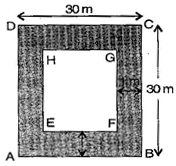Side of square garden without path

= 30 – (1 + 1) = 30 – 2 = 28 m

Now Area of path = Area of ABCD – Area of EFGH

= (AB x AD) – (EF x EH)

= (30 x 30) – (28 x 28)

= 900 – 784

= 116 {tex}{m^2}{/tex}

(ii) Area of remaining portion = 28 x 28 = 784 {tex}{m^2}{/tex}

The cost of planting grass in 1 {tex}{m^2}{/tex}of the garden = Rs. 40

The cost of planting grass in 784 {tex}{m^2}{/tex}of the garden = Rs. 40 x 784 = Rs. 31,360

NCERT Solutions for Class 7 Maths Exercise 11.4

###### Question 6.Two cross roads, each of width 10 m, cut at right angles through the centre of a rectangular park of length 700 m and breadth 300 m and parallel to its sides. Find the area of the roads. Also find the area of the park excluding cross roads. Give the answer in hectares.

Here, PQ = 10 m and PS = 300 m, EH = 10 m and EF = 700 m

And KL = 10 m and KN = 10 m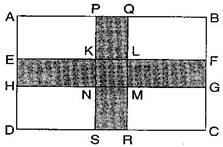= Area of PQRS + Area of EFGH – Area of KLMN

[{tex}\because {/tex} KLMN is taken twice, which is to be subtracted]

= PS x PQ + EF x EH – KL x KN

= (300 x 10) + (700 x 10) – (10 x 10)

= 3000 + 7000 – 100

= 9,900 {tex}{m^2}{/tex}

Area of road in hectares, 1 {tex}{m^2}{/tex}= {tex}\frac{1}{{10000}}{/tex} hectares

{tex}\therefore {/tex} 9,900{tex}{m^2}{/tex}= {tex}\frac{{9900}}{{10000}}{/tex} = 0.99 hectares

Now, Area of park excluding cross roads = Area of park – Area of road

= (AB x AD) – 9,900

= (700 x 300) – 9,900

= 2,10,000 – 9,900

= 2,00,100 {tex}{m^2}{/tex}

= {tex}\frac{{200100}}{{10000}}{/tex} hectares = 20.01 hectares

NCERT Solutions for Class 7 Maths Exercise 11.4

###### Question 7.Through a rectangular field of length 90 m and breadth 60 m, two roads are constructed which are parallel to the sides and cut each other at right angles through the centre of the fields. If the width of each road is 3 m, find:

(i) the area covered by the roads.

(ii) the cost of constructing the roads at the rate of Rs. 110 per{tex}{m^2}{/tex}.

(i) Here, PQ = 3 m and PS = 60 m, EH = 3 m and

EF = 90 m and KL = 3 m and KN = 3 m

= Area of PQRS + Area of EFGH – Area of KLMN

[{tex}\because {/tex} KLMN is taken twice, which is to be subtracted]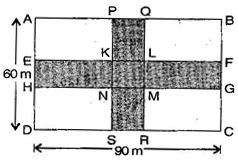= PS x PQ + EF x EH – KL x KN

= (60 x 3) + (90 x 3) – (3 x 3)

= 180 + 270 – 9

= 441 {tex}{m^2}{/tex}

(ii) The cost of 1 {tex}{m^2}{/tex} constructing the roads = Rs. 110

The cost of 441 {tex}{m^2}{/tex} constructing the roads = Rs. 110 x 441 = Rs. 48,510

Therefore, the cost of constructing the roads = Rs. 48,510

NCERT Solutions for Class 7 Maths Exercise 11.4

###### Question 8.Pragya wrapped a cord around a circular pipe of radius 4 cm (adjoining figure) and cut off the length required of the cord. Then she wrapped it around a square box of side 4 cm (also shown). Did she have any cord left? {tex}\left( {{\text{Take }}\pi {\text{ = 3}}{\text{.14}}} \right){/tex}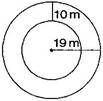Radius of pipe = 4 cm

Wrapping cord around circular pipe = {tex}2\pi r{/tex}

= 2 x 3.14 x 4 = 25.12 cm

Again, wrapping cord around a square = 4 x side

= 4 x 4 = 16 cm

Remaining cord = Cord wrapped on pipe – Cord wrapped on square

= 25.12 – 16 = 9.12 cm

Thus, she has left 9.12 cm cord.

NCERT Solutions for Class 7 Maths Exercise 11.4

###### Question 9.The adjoining figure represents a rectangular lawn with a circular flower bed in the middle. Find: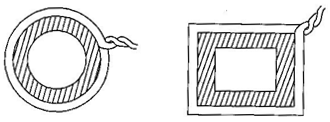(i) the area of the whole land.

(ii) the area of the flower bed.

(iii) the area of the lawn excluding the area of the flower bed.

(iv) the circumference of the flower bed.

Length of rectangular lawn = 10 m, breadth of the rectangular lawn = 5 m

And radius of the circular flower bed = 2 m

(i) Area of the whole land = length x breadth

= 10 x 5 = 50{tex}{m^2}{/tex}

(ii) Area of flower bed = {tex}\pi {r^2}{/tex}

= 3.14 x 2 x 2 = 12.56{tex}{m^2}{/tex}

(iii) Area of lawn excluding the area of the flower bed = area of lawn – area of flower bed

= 50 – 12.56

= 37.44 {tex}{m^2}{/tex}

(iv) The circumference of the flower bed = {tex}2\pi r{/tex}

= 2 x 3.14 x 2 = 12.56 m

NCERT Solutions for Class 7 Maths Exercise 11.4

###### Question 10.In the following figures, find the area of the shaded portions: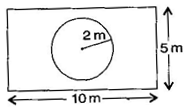(i) Here, AB = 18 cm, BC = 10 cm, AF = 6 cm, AE = 10 cm and BE = 8 cm

Area of shaded portion = Area of rectangle ABCD – (Area of {tex}\Delta {/tex}FAE + area of {tex}\Delta {/tex}EBC)

= (AB x BC) – ({tex}\frac{1}{2}{/tex} x AE x AF + {tex}\frac{1}{2}{/tex} x BE x BC)

= (18 x 10) – ({tex}\frac{1}{2}{/tex} x 10 x 6 + {tex}\frac{1}{2}{/tex} x 8 x 10)

= 180 – (30 + 40)

= 180 – 70 = 110 cm2

(ii) Here, SR = SU + UR = 10 + 10 = 20 cm, QR = 20 cm

PQ = SR = 20 cm, PT = PS – TS = 20 – 10 cm

TS = 10 cm, SU = 10 cm, QR = 20 cm and UR = 10 cm

= Area of square PQRS – Area of {tex}\Delta {/tex}QPT – Area of {tex}\Delta {/tex}TSU – Area of {tex}\Delta {/tex}UQR

= (SR x QR) – {tex}\frac{1}{2}{/tex} x PQ x PT – {tex}\frac{1}{2}{/tex} x ST x SU – {tex}\frac{1}{2}{/tex}

= 20 x 20 – {tex}\frac{1}{2}{/tex} x 20 x 10 – {tex}\frac{1}{2}{/tex} x 10 x 10 – {tex}\frac{1}{2}{/tex} x 20 x 10

= 400 – 100 – 50 – 100 = 150 cm2

NCERT Solutions for Class 7 Maths Exercise 11.4

###### Question 11.Find the area of the equilateral ABCD. Here, AC = 22 cm, BM = 3 cm, DN = 3 cm and BM {tex} \bot {/tex} AC, DN {tex} \bot {/tex} AC.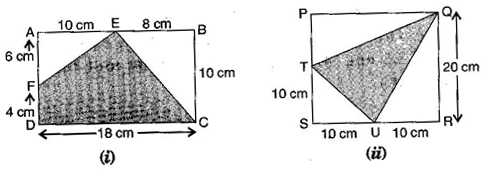Here, AC = 22 cm, BM = 3 cm, DN = 3 cm

Area of quadrilateral ABCDF = Area of {tex}\Delta {/tex}ABC + Area of {tex}\Delta {/tex}ADC

= {tex}\frac{1}{2}{/tex} x AC x BM + {tex}\frac{1}{2}{/tex} x AC x DN

= {tex}\frac{1}{2}{/tex} x 22 x 3 + {tex}\frac{1}{2}{/tex} x 22 x 3

= 3 x 11 + 3 x 11

= 33 + 33

= 66 {tex}c{m^2}{/tex}

Thus, the area of quadrilateral ABCD is{tex}c{m^2}{/tex}.

## NCERT Solutions for Class 7 Maths Exercise 11.4

NCERT Solutions Class 7 Maths PDF (Download) Free from myCBSEguide app and myCBSEguide website. Ncert solution class 7 Maths includes text book solutions from Class 7 Maths Book . NCERT Solutions for CBSE Class 7 Maths have total 15 chapters. 7 Maths NCERT Solutions in PDF for free Download on our website. Ncert Maths class 7 solutions PDF and Maths ncert class 7 PDF solutions with latest modifications and as per the latest CBSE syllabus are only available in myCBSEguide.

## CBSE app for Students

To download NCERT Solutions for Class 7 Maths, Social Science Computer Science, Home Science, Hindi English, Maths Science do check myCBSEguide app or website. myCBSEguide provides sample papers with solution, test papers for chapter-wise practice, NCERT solutions, NCERT Exemplar solutions, quick revision notes for ready reference, CBSE guess papers and CBSE important question papers. Sample Paper all are made available through the best app for CBSE students and myCBSEguide website.### Test Generator

Create question paper PDF and online tests with your own name & logo in minutes.### myCBSEguide

Question Bank, Mock Tests, Exam Papers, NCERT Solutions, Sample Papers, Notes

### 10 thoughts on “NCERT Solutions for Class 7 Maths Exercise 11.4”

1. nice

2. Good

3. Good

4. This is so good……as my school teacher is not teaching nice at all…..I am always searching on this NCERT solutions i am getting all the answers…….nd this is easy to understand also…..

5. thanks.love it

6. Thanks.love it

7. Ur very helpful for me